Win up to 100% scholarship on Aakash BYJU'S JEE/NEET courses with ABNAT Win up to 100% scholarship on Aakash BYJU'S JEE/NEET courses with ABNAT

# JEE Main 2021 Maths Paper With Solutions Feb 24 Shift 2

Download the JEE Main 2021 Maths question paper for Feb 24 shift 2 from this page. Students can use this paper which contains detailed solutions prepared by experts as study material to further test their knowledge and strengthen their preparation for the upcoming exam. By solving the questions in the given paper students will further develop adept problem-solving and time management skills that will help them to perform excellently in the entrance exam.

Students should go through the JEE Main 2021 Maths question paper before writing the main exam. This will also help in the quick revision of the important topics and students will also gain a much better idea about the exam pattern, difficulty level of the question and the marking scheme. The Maths question paper is a perfect resource that will allow candidates to practice solving different types of problems more accurately and boost their preparation for the main exam.

JEE Main 2021 24th February Shift 2 Maths Question Paper

SECTION A

Question 1: Let a, b∈R. If the mirror image of the point P(a, 6, 9) with respect to the line (x – 3)/7 = (y – 2)/5 = (z – 1)/(-9) is (20, b, -a, -9), then |a + b| is equal to:

a. 86
b. 88
c. 84
d. 90

P(a, 6, 9), Q (20, b, -a-9)

Mid point of PQ=((a+20)/2, (b+6)/2, -a/2) lie on the line.

$$\begin{array}{l}\left ( \frac{\frac{a+20}{2}-3}{7} \right )= \left ( \frac{\frac{b+6}{2}-2}{5} \right )=\left ( \frac{\frac{-a}{2}-1}{-9} \right )\end{array}$$

=> (a + 20 – 6)/14 = (b + 6 – 4)/10 = (-a – 2)/(-18)

=> (a + 14)/14 = (a + 2)/18

=> 18a + 252 = 14a + 28

=> 4a = -224

a = -56

(b + 2)/10 = (a + 2)/18

=> (b + 2)/10 = (-54)/18

=>(b + 2)/10 = -3

=> b = -32

|a + b| = |-56 – 32|

= 88

Question 2: Let f be a twice differentiable function defined on R such that f(0) = 1, f'(0) = 2 and f'(x) ≠ 0 for all x∈R. If |f(x) f'(x) f'(x) f”(x)| = 0, for all x∈R, then the value of f(1) lies in the interval:

a. (9, 12)
b. (6, 9)
c. (3, 6)
d. (0, 3)

Given f(x) f”(x) – f'(x)2 =0

Let h(x) = f(x)/f'(x)

Then h'(x) = 0

⇒ h(x) = k

⇒ f(x)/f'(x) = k

⇒ f(x) = k f’(x)

⇒ f(0) = k f'(0)

⇒ k = 1/2

Now, f(x) = ½ f'(x)

⇒∫ 2dx = ∫ f'(x)/f(x) dx

⇒ 2x = ln |f(x)| + C

As f(0) = 1 ⇒ C = 0

⇒ 2x = ln |f(x)|

⇒ f(x) = ±e2x

As f(0) = 1 ⇒ f(x) = e2x

∴ f(1) = e2 ≈ 7.38

Question 3: A possible value of tan (¼ sin-1 √63/8) is:

a. 1/(2√2)
b. 1/√7
c. √7 – 1
d. 2√2 – 1

tan (¼ sin-1 √63/8)

Let sin-1(√63/8) = θ

sin θ = √63/8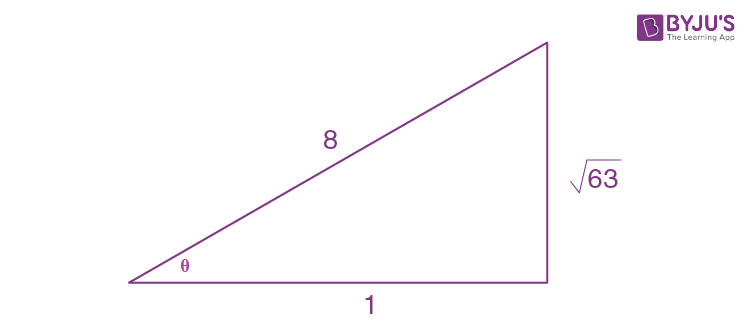cos θ = 1/8

2 cos2(θ/2) – 1 = 1/8

⇒ cos2 θ/2 = 9/16

cos θ/2 = 3/4

⇒(1- tan2 θ/4 )/(1 + tan2 θ/4) = 3/4

tan θ/4 = 1/√7

Question 4: The probability that two randomly selected subsets of the set {1, 2, 3, 4, 5} have exactly two elements in their intersection, is:

a. 65/27
b. 135/29
c. 65/28
d. 35/27

Let A and B be two subsets.

For each x∈{1,2,3,4,5} , there are four possibilities:

x ∈ A∩B, x∈ A’∩B, x ∈ A∩B’, x ∈ A’∩B’

So, the number of elements in sample space =45

Required probability

= (5C2 × 33)/45

= (10×27)/210

= 135/29

Question 5: The vector equation of the plane passing through the intersection of the planes

$$\begin{array}{l}\vec{r}.(\hat{i}+\hat{j}+\hat{k}) = 1\end{array}$$
and
$$\begin{array}{l}\vec{r}.(\hat{i}-2\hat{j}) = -2\end{array}$$
and the point (1, 0, 2) is:

a.

$$\begin{array}{l}\vec{r}.(\hat{i}-7\hat{j}+3\hat{k}) = \frac{7}{3}\end{array}$$

b.
$$\begin{array}{l}\vec{r}.(\hat{i}+7\hat{j}+3\hat{k}) = 7\end{array}$$

c.
$$\begin{array}{l}\vec{r}.(3\hat{i}+7\hat{j}+3\hat{k}) = 7\end{array}$$

d.
$$\begin{array}{l}\vec{r}.(\hat{i}+7\hat{j}+3\hat{k}) = \frac{7}{3}\end{array}$$

Family of planes passing through the intersection of planes is

$$\begin{array}{l}(\vec{r}.(\hat{i}+\hat{j}+\hat{k})-1)+\lambda (\vec{r}.(\hat{i}-2\hat{j})+2) =0\end{array}$$

The above curve passes through

$$\begin{array}{l}\hat{i}+2\hat{k}\end{array}$$
,

(3 − 1) + λ(1 + 2) = 0

⇒ λ = -2/3

Hence, equation of plane is

$$\begin{array}{l}3(\vec{r}.(\hat{i}+\hat{j}+\hat{k})-1)-2(\vec{r}.(\hat{i}-2\hat{j})+2)= 0\end{array}$$

$$\begin{array}{l}\vec{r}.(\hat{i}+7\hat{j}+3\hat{k}) = 7\end{array}$$

TRICK: Only option (2) satisfies the point (1, 0, 2)

Question 6: If P is a point on the parabola y = x2 + 4 which is closest to the straight line y = 4x − 1, then the co-ordinates of P are :

a. (–2, 8)
b. (1, 5)
c. (3, 13)
d. (2, 8)

Tangent at P is parallel to the given line.

dy/dx|P = 4

⇒2x1 = 4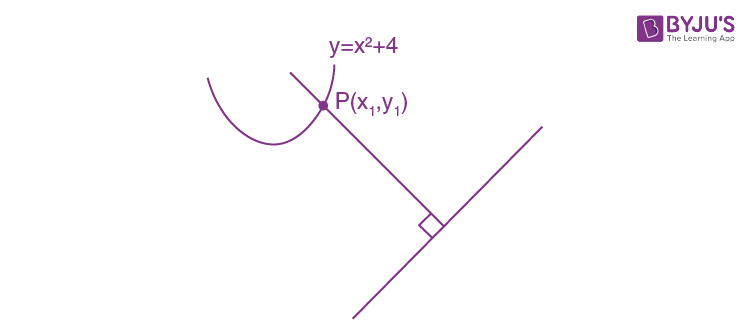⇒x1 = 2

Required point is (2, 8)

Question 7: Let a, b, c be in arithmetic progression. Let the centroid of the triangle with vertices (a, c), (2, b) and (a, b) be (10/3, 7/3). If α, β are the roots of the equation ax2 + bx + 1 = 0, then the value of α2 + β2 – αβ is:

a. 71/256
b. -69/256
c. 69/256
d. -71/256

2b = a + c

(2a + 2)/3 = 10/3 and (2b + c)/3 = 7/3

⇒ a = 4

2b + c = 7, 2b – c = 4 },solving

b = 11/4 and c = 3/2

∴ Quadratic equation is 4x2+ (11/4)x + 1 = 0

∴ The value of (α + β)2 – 3αβ = (121/256) – (¾)

= -71/256

Question 8: The value of the integral,

$$\begin{array}{l}\int_{1}^{3}\left [ x^{2}-2x-2 \right ]dx\end{array}$$
where [x] denotes the greatest integer less than or equal to x , is :

a. –4
b. –5
c. -√2 – √3 – 1
d. -√2 – √3 + 1

$$\begin{array}{l}I = \int_{1}^{3}-3 dx + \int_{1}^{3}\left [ (x-1)^{2} \right ]dx\end{array}$$

Put x – 1 = t ; dx = dt

$$\begin{array}{l}I = (-6) + \int_{0}^{2}\; \; \; \; \; \;[t^{2}]dt\end{array}$$
$$\begin{array}{l}I = -6 + \int_{0}^{2}\: \: \:0dt + \int_{1}^{\sqrt{2}}\: \: \:1dt + \int_{\sqrt{2}}^{\sqrt{3}}\: \: \:2dt + \int_{\sqrt{3}}^{2}\: \: \: 3dt\end{array}$$

I = -6 + (√2 – 1) + 2√3 – 2√2 + 6 – 3√3

I = -1 – √2 – √3

Question 9: Let f: R → R be defined as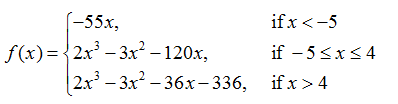Let A = {x ∈ R ∶ f is increasing}. Then A is equal to :

a. (-5, -4) ∪ (4,∞)
b. (-5, ∞)
c. (-∞, -5) ∪ (4, ∞)
d. (-∞, -5) ∪ (-4, ∞)

f’(x) = {-55 ; x< -5 6(x2 – x – 20) ; -5 < x < 4 6(x2– x – 6) ; x > 4

⇒ f’(x) = {-55 ; x< -5 6(x – 5)(x + 4) ; –5 < x < 4 6(x – 3)(x + 2) ; x > 4

Hence, f(x) is monotonically increasing in (-5, -4) ∪ (4, ∞)

Question 10: If the curve y = ax2 + bx + c,x ∈ R passes through the point (1, 2) and the tangent line to this curve at origin is y = x, then the possible values of a, b, c are:

a. a = 1, b = 1, c = 0
b. a = -1, b = 1, c = 1
c. a = 1, b = 0, c = 1
d. a = 1/2, b = 1/2 ,c = 1

2 = a + b + c

dy/dx = 2ax + b, (dy/dx)(0,0) = 1

⇒ b = 1 and a + c = 1

Since (0, 0) lies on curve,

∴ c = 0, a = 1

TRICK: (0, 0) lies on the curve. Only option (1) has c = 0

Question 11: The negation of the statement ~p∧(p∨q) is∶

a. ~p∧q
b. p∧~q
c. ~p∨q
d. p∨∼q

Negation of ~p∧(p∨q) is

∼[~p∧(p∨q)]

≡ p∨ ∼(p∨q)

≡ p∨(∼p∧∼q)

≡ (p∨∼p)∧(p∨∼q)

≡ T∧(p∨∼q), where T is tautology.

≡ p∨∼q

Question 12: For the system of linear equations: x − 2y = 1, x − y + kz = −2, ky + 4z = 6,k ∈ R

Consider the following statements:

(A) The system has unique solution if k ≠ 2,k ≠ −2.

(B) The system has unique solution if k = -2.

(C) The system has unique solution if k = 2.

(D) The system has no-solution if k = 2.

(E) The system has infinite number of solutions if k ≠ -2.

Which of the following statements are correct?

a. (B) and (E) only
b. (C) and (D) only
c. (A) and (D) only
d. (A) and (E) only

x -2y + 0.z = 1

x – y + kz = -2

0.x + ky + 4z = 6

∆ =

$$\begin{array}{l}\begin{vmatrix} 1 & -2 & 0\\ 1& -1 & k\\ 0& k & 4 \end{vmatrix}\end{array}$$

= 4 – k2

For unique solution, 4 – k2 ≠ 0

k ≠ ±2

For k = 2,

x – 2y + 0.z =1

x – y + 2z = -2

0.x + 2y + 4z = 6

Δx =

$$\begin{array}{l}\begin{vmatrix} 1 & -2 & 0\\ -2& -1 & 2\\ 6& 2 & 4 \end{vmatrix}\end{array}$$

= -8 + 2 (-20)

⇒Δx= -48 ≠ 0

For k = 2,Δx ≠ 0

So, for k = 2, the system has no solution.

Question 13: For which of the following curves, the line x + √3y = 2√3 is the tangent at the point (3√3/2, 1/2)?

a. x2 + 9y2 = 9
b. 2x2 – 18y2 = 9
c. y2 = x/(6√3)
d. x2 + y2 = 7

Tangent to x2 + 9y2 = 9 at point (3√3/2, 1/2) is x(3√3)/2 + 9y(1/2) = 9

⇒3√3 x + 9y = 18

⇒x +√3 y = 2√3

⇒ Option (a) is true.

Question 14: The angle of elevation of a jet plane from a point A on the ground is 600. After a flight of 20 seconds at the speed of 432 km/hour, the angle of elevation changes to 300. If the jet plane is flying at a constant height, then its height is:

a. 1200√3 m
b. 1800√3 m
c. 3600√3 m
d. 2400√3 m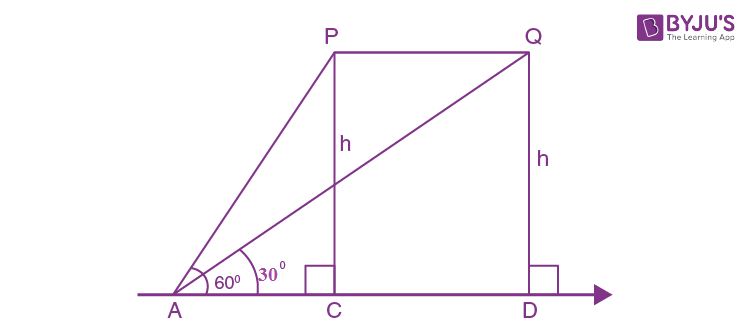v = 432 × 1000/(60×60) m/sec

= 120 m/sec

Distance PQ = v × 20 = 2400 m

In ΔPAC

tan 600 = h/AC

⇒ AC = h/(√3)

In ΔAQD

⇒√3 h = h/√3 + 2400

⇒2h/√3 = 2400

⇒ h = 1200√3 m

Question 15: For the statements p and q, consider the following compound statements:

(a) (~q∧(p→q)) → ~p

(b) ((p∨q))∧~p) → q

Then which of the following statements is correct?

a. (a) is a tautology but not (b)
b. (a) and (b) both are not tautologies
c. (a) and (b) both are tautologies
d. (b) is a tautology but not (a)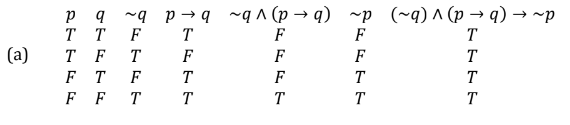(a) is tautology.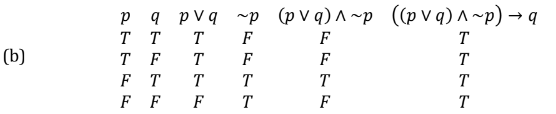(b) is tautology.

∴ (a) and (b) both are tautologies.

Question 16: Let A and B be 3×3 real matrices such that A is symmetric matrix and B is skew-symmetric matrix. Then the system of linear equations (A2B2 − B2A2)X = O, where X is a 3×1 column matrix of unknown variables and O is a 3×1 null matrix, has:

a. a unique solution
b. exactly two solutions
c. infinitely many solutions
d. no solution

AT = A, BT = –B

Let A2B2 − B2A2= P

PT = (A2B2 – B2A2)T

= (A2B2)T – (B2A2)T

= (B2)T (A2)T– (A2)T (B2)T

= B2A2 – A2B

⇒ P is a skew-symmetric matrix.

$$\begin{array}{l}\begin{bmatrix} 0 & a & b\\ -a & 0 &c \\ -b &-c & 0 \end{bmatrix}\begin{bmatrix} x & y & z \end{bmatrix}=\begin{bmatrix} 0 & 0 & 0 \end{bmatrix}\end{array}$$

∴ ay + bz = 0 …(1)

–ax + cz = 0 …(2)

–bx – cy =0 …(3)

From equation (1), (2), (3)

Δ = 0 and Δ1 = Δ2= Δ3 = 0

∴ System of equations has infinite number of solutions.

Question 17: If n ≥ 2 is a positive integer, then the sum of the series n+1C2 + 2(2C2 + 3C2 + 4C2 + …. + nC2) is

a. n(n + 1)2 (n + 2)/12
b. n(n – 1)(2n + 1)/6
c. n(n + 1)(2n + 1)/6
d. n(2n + 1)(3n + 1)/6

2C2 = 3C3

Let S = 3C3 + 3C2 + ……. + nC2 = n+1C3 (∵ nCr + nCr–1 = n+1Cr)

n+1C2 + n+1C3 + n+1C3

= n+2C3 + n+1C3

=(n + 2)!/3!(n – 1)! + (n + 1)!/3!(n – 2)!

=(n + 2)(n + 1)n/6 + (n + 1)(n)(n – 1)/6 = (n(n + 1)(2 + 1))/6

TRICK : Put n = 2 and verify the options.

Question 18: If a curve y = f(x) passes through the point (1, 2) and satisfies xdy/dx + y = bx4, then for what value of b,

$$\begin{array}{l}\int_{1}^{2}f(x)dx = \frac{62}{5}\end{array}$$

a. 5
b. 62/5
c. 31/5
d. 10

dy/dx + y/x = bx3

I.F. =

$$\begin{array}{l}e^{\int \frac{dx}{x}}\end{array}$$

= x

∴ yx = ∫bx4 dx = bx5/5 + c

The above curve passes through (1, 2).

2 = b/5 + c

Also,

$$\begin{array}{l}\int_{1}^{2}(\frac{bx^{4}}{5}+\frac{c}{x})dx=\frac{62}{5}\end{array}$$

⇒ (b/25) ×32 + c ln 2 – b/25 = 62/5

⇒ c = 0 and b = 10

Question 19: The area of the region: R{(x, y): 5x2 ≤ y ≤ 2x2 + 9} is:

a. 9√3 square units
b. 12√3 square units
c. 11√3 square units/
d. 6√3 square units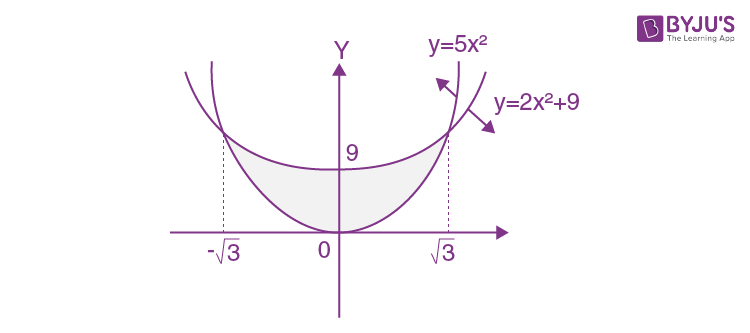Required area

$$\begin{array}{l}2\int_{0}^{\sqrt{3}}\: \: \:(2x^{2}+9-5x^{2})dx\end{array}$$

=

$$\begin{array}{l}2\int_{0}^{\sqrt{3}}\: \: \:(9-3x^{2})dx\end{array}$$

$$\begin{array}{l}2\left | 9x-x^{3} \right |_{0}^{\sqrt{3}}\end{array}$$

= 12√3

Question 20: Let f(x) be a differentiable function defined on [0,2] such that f’(x) =f ‘(2 – x) for all x∈(0, 2), f(0) = 1 and f(2) = e2. Then the value of

$$\begin{array}{l}\int_{0}^{2}f(x)dx\end{array}$$
is:

a. 1 + e2
b. 1 – e2
c. 2(1 – e2)
d. 2(1+e2)

f'(x) = f'(2 – x)

On integrating both sides, we get

f(x) = -f(2 – x)+c

Put x = 0

f(0) + f(2) = c

⇒ c = 1+ e2

⇒ f(x) + f(2 – x) = 1 + e2

I =

$$\begin{array}{l}\int_{0}^{2}f(x)dx= \int_{0}^{1}(f(x) + f(2-x))dx\end{array}$$

= 1+e2

Section B

Question 1: The number of the real roots of the equation (x + 1)2 + |x – 5| = 27/4 is ___.

For x ≥ 5,

(x + 1)2+ (x – 5) = 27/4

⇒ x2 + 3x – 4 = 27/4

⇒ x2 + 3x – 43/4 = 0

⇒ 4x2 + 12x – 43 = 0

x = (-12 ± √(144 + 688))/8

x = (-12 ± √832)/8

= (-12 ± 28.8)/8

= (-3 ± 7.2)/2

= (-3 + 7.2)/2, (-3 – 7.2)/2 (therefore, no solution)

For x < 5,

(x + 1)2– (x – 5) = 27/4

⇒ x2 + x + 6 – 27/4=0

⇒ 4x2 + 4x – 3=0

x = (-4 ± √(16 + 48))/8

x = (-4 ± 8)/8

⇒ x = -12/8, 4/8

∴ 2 real roots.

Question 2: The students S1, S2,… S10 are to be divided into 3 groups A, B, and C such that each group has at least one student and the group C has at most 3 students. Then the total number of possibilities of forming such groups is ___.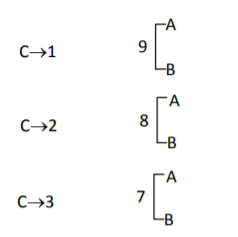Number of ways

= 10C1 [29 – 2] + 10C2 [28– 2] + 10C3 [27 – 2]

= 27 [10C1×4 + 10C2 ×2 + 10C3] – 20 – 90 – 240

= 128 [40 + 90 + 120 ] – 350

= (128 × 250) – 350

= 10 

= 31650

Question 3: If a + α = 1, b + β = 2 and af(x) + αf(1/x) = bx + β/2, x ≠ 0 then the value of the expression [f(x) + f(1/x) ]/(x + 1/x)

af(x) + αf(1/x) = bx + β/x …(i)

Replace x by 1/x

af(1/x) + αf(x) = b/x + βx …(ii)

(i) + (ii)

(a + α)[f(x) + f(1/x) ]

= (x + 1/x)(b + β)

⇒ ( f(x) + f(1/x))/(x + 1/x)

= 2/1

= 2

Question 4: If the variance of 10 natural numbers 1,1,1,…,1,k is less than 10, then the maximum possible value of k is ________.

$$\begin{array}{l}\sigma ^{2} = \frac{\Sigma x^{2}}{n} – \left (\frac{\Sigma x}{n} \right )^{2}\end{array}$$

$$\begin{array}{l}\sigma ^{2} = \frac{9+k^{2}}{10} – \left (\frac{9+k}{10} \right )^{2}< 10\end{array}$$

⇒ 10(9 + k2) – (81 + k2 + 18k) < 1000

⇒ 90 + 10k2 – k2 – 18k – 81 < 1000

⇒ 9k2– 18k + 9 < 1000

⇒ (k – 1)2 < 1000/9

⇒ k – 1< (10√10)/3

⇒ k < (10√10)/3 + 1

Maximum possible integral value of k is 11.

Question 5: Let λ be an integer. If the shortest distance between the lines x – λ = 2y – 1 = -2z and x = y + 2λ = z – λ is √7/2√2, then the value of |λ| is __

(x – λ)/1 = (y – 1/2)/(1/2) = z/(-1/2)

(x – λ)/2 = (y-1/2)/1 = z/(-1) …(1) Point on line = (λ, 1/2, 0)

x/1 = (y + 2λ)/1 = (z – λ)/1 …(2) Point on line = (0, -2λ, λ)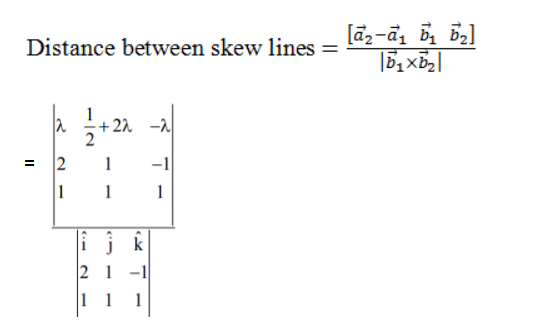= |-5λ – 3/2|/√14

= √7/(2√2) (Given)

⇒ |10λ + 3| = 7

⇒ λ = -1 as λ is an integer.

⇒ |λ| = 1

Question 6: If i = √-1. If [(-1 + i√3)21/(1 – i)24 + (1 + i√3)21/(1 + i)24 ] = k, and n = [|k| ] be the greatest integral part of |k|. Then

$$\begin{array}{l}\sum_{j=0}^{n+5}(j+5)^{2} – \sum_{j=0}^{n+5}(j+5)\end{array}$$
is equal to ___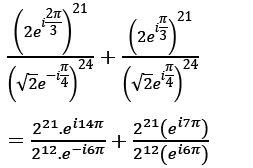= 29 ei(20π) +29 eiπ

= 29 + 29 (-1)

= 0 = k

∴ n = 0

(j=0)5 (j + 5)2– ∑(j=0)5 (j + 5)

= [52 + 62 + 72 + 82 + 92 + 102 ] – [5 + 6 + 7 + 8 + 9 + 10]

= [(12+ 22 +… + 102) – (12 + 22+ 32+ 42) ] – [(1 + 2 + 3 + … + 10) – (1 + 2 + 3 + 4) ]

= (385 – 30) -[55 – 10]

= 355 – 45

= 310

Question 7: Let a point P be such that its distance from the point (5, 0) is thrice the distance of P from the point (-5, 0). If the locus of the point P is a circle of radius r, then 4r2 is equal to ___.

Let P be (h, k), A(5, 0) and B(-5, 0)

Given PA = 3PB

⇒ PA2 = 9PB2

⇒ (h-5)2 + k2 = 9[(h + 5)2 + k2]

⇒ 8h2 + 8k2 + 100h + 200 = 0

∴ Locus of P is x2 + y2 + (25/2)x + 25 = 0

Centre = (-25/4, 0)

∴ r2 = (-25/4)2 – 25

= 625/16 – 25

= 225/16

∴4r2 = 4×225/16

= 225/4

= 56.25

Question 8: For integers n and r, let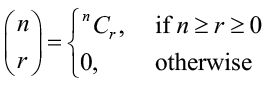The maximum value of k for which the sum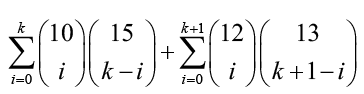exists, is equal to ____.

(1+x)10 = 10C0 + 10C1x + 10C2x2 + …… + 10C10x10

(1+x)15= 15C0 + 15C1x +15C2x2 + …… + 15Ck-1xk-1 + 15Ck+1xk+1 + …15C15x15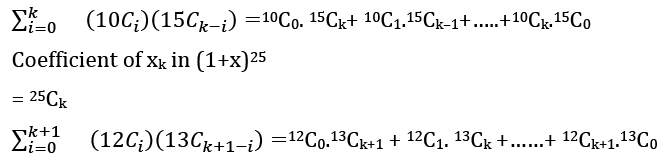Coefficient of xk+1 in (1+x)25

= 25Ck+1

25Ck + 25Ck+1 = 26Ck+1

For maximum value

as per given, k can be as large as possible.

Question 9: The sum of first four terms of a geometric progression (G.P.) is 65/12 and the sum of their respective reciprocals is 65/18. If the product of first three terms of the G.P. is 1, and the third term is α then 2α is____

Let terms of GP are a, ar, ar2, ar3

a + ar + ar2 + ar3 = 65/12 …….(1)

1/a + 1/ar + 1/(ar2) + 1/ar3) = 65/18

⇒1/a (r3 + r2 + r + 1)/r3) = 65/18 …….(2)

(1)/(2), we get

a2r3 = 18/12

= 3/2

Also, a3 r3 = 1

⇒ a(3/2) = 1

⇒a = 2/3

(4/9) r3 = 3/2

⇒ r3 = 33/23

⇒ r = 3/2

α = ar2

= 2/3.(3/2)2 = 3/2

∴2α = 3

Question 10: If the area of the triangle formed by the positive x-axis, the normal and the tangent to the circle (x – 2)2+ (y – 3)2 = 25 at the point (5, 7) is A, then 24A is equal to ___.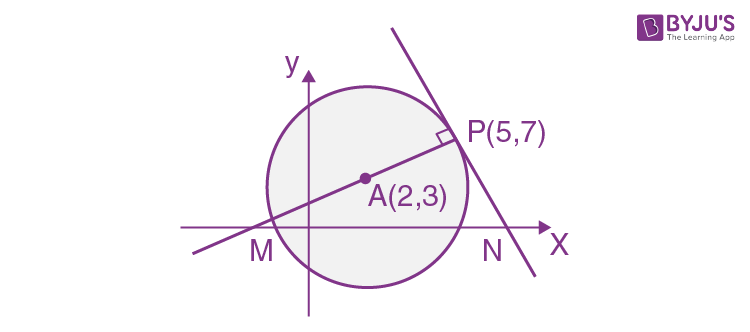Equation of normal at P is

(y – 7) = ((7 – 3)/(5 – 2))(x – 5)

⇒ 3y – 21 = 4x – 20

⇒ 4x – 3y + 1 = 0

⇒ M is (-1/4, 0)

Equation of tangent at P is

(y – 7) = (-¾) (x – 5)

⇒ 4y – 28 = -3x + 15

⇒ 3x + 4y = 43

⇒ N is (43/3, 0)

The question is wrong. The normal cuts at a point on the negative axis.